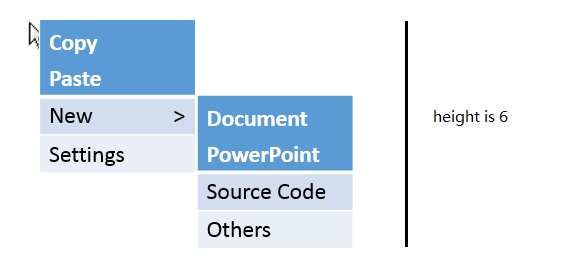题意分析算法分析

• `row`: 表示一行选项

• `section`: 由至少一行`row`构成，其中`row`的顺序可以自由排列

• `panel`: 由至少一个`section`构成，其中`section`的顺序可以自由排列

`panel`只包含有1个`section``section`包含有 s`row`，记为`R1`..`Rn`，但是只有`R1`有子菜单，且长度为 r

`panel`只包含有1个`section``section`包含有 s`row`，记为`R1`..`Rn`，其中`R1`有长度为 r1 的子菜单，`R2`有长度为 r2 的子菜单，且 r1r2

`panel`只包含有2个`section`，记为`S1`,`S2`

`S1`包含有 s1`row`，且展开这些`row`使得`S1`最少延伸到 sr1 行(即从`S1`的第 1 行开始计算，其展开的子菜单最长延伸到第 sr1 行)，令 ∆1 = sr1 - s1。举个例子：

``````+-----------+
| other sec |
+-----------+                 -   -
|   S1 r1   |                 ^   ^
+-----------+-----------+     |   |
|   S1 r2  >|           |     |   s1
+-----------+-----------+     |   |
|   S1 r3   |           |    sr1  v
+-----------+-----------+     |   -
| other sec |           |     |   |
+-----------+-----------+     |   ∆1
|           |     v   |
+-----------+     -   -
``````

`S2`包含有 s2`row`，且展开这些`row`使得`S2`最少延伸到 sr2 行，令 ∆2 = sr2 - s2

``````对于row和section，我们要将子菜单长度与本体长度差值大的靠前放置。
``````

1. `row`：递归处理出每一个`row`的子菜单长度。

2. `section`: 将`section`内的`row`进行排序，得到`section`的最优长度方案，记录其本体长度和子菜单长度。

3. `panel`：将`panel`内的`section`进行排序，得到`panel`的最优长度。

``````struct row {
int childId;          // 子菜单指针
int expandLength;     // 子菜单长度
row():childId(-1),expandLength(0) {};
row(int _id):childId(_id),expandLength(0) {};
};

struct section {
vector< row > rows;   // 包含的row情况
int selfLength;       // 自身的长度 s1
int expandLength;     // 展开子菜单之后的长度 sr1
int delta;            // 子菜单之后的长度与自身的差值 ∆
section():selfLength(0),expandLength(0),delta(0) {};
};

struct panel {
vector< section > sections; // 包含的section情况
vector< int > rowIds;       // 读入时该panel内的rowId
};
``````

``````panel panels[ MAXN ];

// 读入每一个panel的情况
int n;
cin >> n;
int id, numOfIds;
for (int i = 0; i <= n; ++i) {
cin >> numOfIds;
while (numOfIds--) {
cin >> id;
if (id == 0) ++numOfIds;
panels[i].rowIds.push_back(id);
}
dealPanel( panels[i] );    // 处理panel的情况
}
``````

``````void dealPanel(panel &p) {
if ( (int) p.rowIds.size() == 0 ) return ;

p.sections.push_back(section());
int sectionId = 0;

// 加入一个末尾0，方便处理最后一个section
p.rowIds.push_back(0);

// 依次出每一个section
for (int i = 0; i != (int) p.rowIds.size(); ++i)
if (p.rowIds[i] != 0) {
p.sections[ sectionId ].rows.push_back( row(p.rowIds[i]) );
}   else {
// 新的section
p.sections.push_back(section());
sectionId++;
}
return ;
}
``````

``````bool sortByExpandLength(row x, row y) {
return x.expandLength > y.expandLength;
}

bool sortByDelta(section x, section y) {
return x.delta > y.delta;
}

int getExpandLength(panel &p)
{

// ret初始化为0，ret表示该panel的最小展开长度
int ret = 0;

// 枚举每一个section
for (int i = 0; i != (int) p.sections.size(); ++i)
{

// 处理该section内的每一个row的子菜单
for (int j = 0; j != (int) p.sections[i].rows.size(); ++j)
p.sections[i].rows[j].expandLength = getExpandLength( panels[ p.sections[i].rows[j].childId ] );

// 根据row的expandLength对section内的row进行排序
sort(p.sections[i].rows.begin(), p.sections[i].rows.end(), sortByExpandLength);

// 处理得到section的值
p.sections[i].selfLength = (int) p.sections[i].rows.size();
p.sections[i].expandLength = p.sections[i].selfLength; // 展开值至少为selfLength

// 枚举每一个row，找到展开值最大的作为section的展开值
for (int j = 0; j != (int) p.sections[i].rows.size(); ++j)
if (p.sections[i].expandLength < j + p.sections[i].rows[j].expandLength)
p.sections[i].expandLength = j + p.sections[i].rows[j].expandLength;

// 计算section的∆值
p.sections[i].delta = p.sections[i].expandLength - p.sections[i].selfLength;

// 累加panel内所有的row行数作为最小的ret
ret += p.sections[i].selfLength;
}

// 根据section的∆值进行排序
sort(p.sections.begin(), p.sections.end(), sortByDelta);

// now记录当前section的起始行数
int now = 0;

// 枚举每一个section，找到展开值最大的作为panel的展开值
for (int i = 0; i != (int) p.sections.size(); ++i)
{
if (ret < now + p.sections[i].expandLength)
ret = now + p.sections[i].expandLength;

// 累加为下一个section的起始行数
now += p.sections[i].selfLength;
}

return ret;
}
``````

``````cout << getExpandLength( panels ) << endl;
``````

结果分析

• 根据题解来看，如果一个panel中有多个row有子菜单，那么其“长度”应该是由那些使得展开row最长的情况来记录，题目要求的是重新安排row和section，使得这些最长的情况最短。不知我理解的是否确切？

• 添加评论